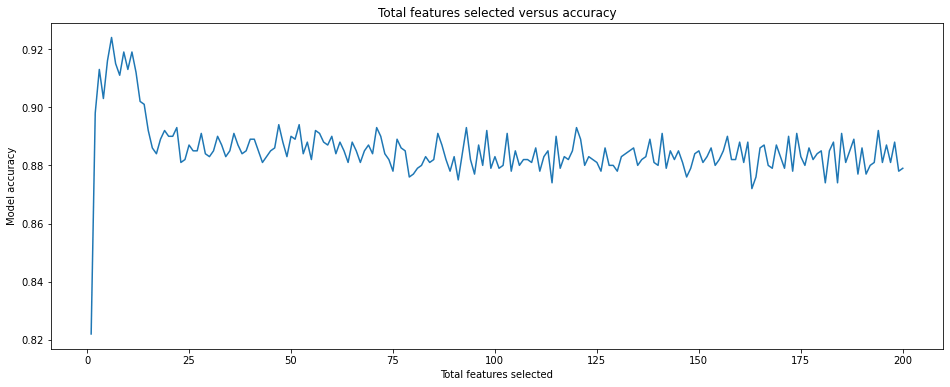Picture by Tine Ivanic, Unsplash.

Something which often confuses non data scientists is that too many features can be a bad thing for a model. It does sound logical that including more features and data might make a model better. However, many features turn out to be uninformative and can actually mislead machine learning models and reduce their performance.

Therefore, one of the steps you’ll likely go through when building a larger model is a process aimed at taking out the irrelevant features that don’t add anything to improve the model’s performance. This process is known as feature selection and is commonly tackled using a technique called Recursive Feature Elimination or RFE.

Recursive Feature Elimination is quite a clever solution to the problem, I think. Recursion is the process of repeating a process multiple times. In the case of RFE, the process is that a separate model is trained and each time different features are taken away. While this process takes place, we observe the impact this has upon the model’s accuracy to determine the optimum set of features to use for the maximum results. Here’s how to use it.

For this project we’ll be using Pandas for data manipulation, Matplotlib for plotting, and a number of different packages from Scikit-Learn, including RFECV for the Recursive Feature Elimination. Load up the packages and install anything you don’t have by entering `pip3 install package-name` into your terminal.

``````import pandas as pd
import matplotlib.pyplot as plt
from sklearn.datasets import make_classification
from sklearn.model_selection import StratifiedKFold
from sklearn.feature_selection import RFECV
from sklearn.datasets import make_classification
from sklearn.tree import DecisionTreeClassifier
``````
``````pd.set_option('max_columns', 6)
``````

### Create a high dimension data set

For this project we’ll create our own synthetic data set specifically engineered so it has a high number of dimensions. We’ll use the `make_classification()` method from Scikit-Learn to do this. We’ll define 1000 samples with 200 features, of which 6 will be informative and useful to the model, and 10 with be uninformative or redundant. We’ll make this a simple binary classification problem.

``````X, y = make_classification(n_samples=1000,
n_features=200,
n_informative=6,
n_redundant=10,
n_classes=2,
random_state=0
)
``````

Once that’s created, we’ll save the resulting Numpy arrays to Pandas dataframes and then print out the shape of the dataframes and check to see how balanced the target variable is within the `y` data set by using the ```value_counts() ``` function.

``````X = pd.DataFrame(X)
y = pd.Series(y)

print(X.shape, y.shape)
``````
``````(1000, 200) (1000,)
``````
``````y.value_counts()
``````
``````0    504
1    496
dtype: int64
``````

### Fit a Recursive Feature Elimination model

Next we’ll use the `RFECV()` feature selection tool in Scikit-Learn to identify the optimum number of features to use in our model to obtain the highest accuracy score. To use this we pass in another Scikit-Learn classifier model to use as the `estimator`. I’ve used `DecisionTreeClassifier()`.

As `RFECV()` also handles cross-validation, I’ve set this to use the `StratifiedKFold()` method and have selected 10 rounds, with accuracy used as my scoring metric. Once that’s set up, I’ve then fit it to my `X` and `y` data.

``````rfecv = RFECV(estimator=DecisionTreeClassifier(),
step=1,
cv=StratifiedKFold(10),
scoring='accuracy')
rfecv.fit(X, y)
``````
``````RFECV(cv=StratifiedKFold(n_splits=10, random_state=None, shuffle=False),
estimator=DecisionTreeClassifier(), scoring='accuracy')
``````

### Examine the output

The `rfecv` object contains five attributes in its output: `n_features_` contains the number of features selected via cross-validation; `support_` contains a mask array of the selected features; `ranking_` contains the feature ranking of the best features; `grid_scores_` contains the cross-validation scores, and `estimators_` is an object contain the estimator we used to reduce dimensionality, i.e. `DecisionTreeClassifier()`. If you print out the `rfecv.n_features_` value you’ll see that this has correctly determined there are 5 informative features in the data.

``````print("Optimum number of features: %d" % rfecv.n_features_)
``````
``````Optimum number of features: 6
``````

To visualise how the model’s performance drops off when you don’t reduce the number of features we can plot the number of features used in the model versus the model accuracy. As you can see, more features isn’t always better, and the model’s accuracy quickly drops off when uninformative features start racking up.

``````plt.figure( figsize=(16, 6))
plt.title('Total features selected versus accuracy')
plt.xlabel('Total features selected')
plt.ylabel('Model accuracy')
plt.plot(range(1, len(rfecv.grid_scores_) + 1), rfecv.grid_scores_)
plt.show()
``````### Identifying the features RFE selected

As we saw above, the `rfecv` object contains some hidden data attributes that we can extract to identify which features the `DecisionTreeClassifier()` identified from our data set as those which were informative. To extract these we’ll create a dataframe, loop through the data and append a new row to the dataframe containing the feature index number, the support level and the ranking. To extract only the informative ones we can then use `df_features[df_features['support']==True]`.

``````df_features = pd.DataFrame(columns = ['feature', 'support', 'ranking'])

for i in range(X.shape):
row = {'feature': i, 'support': rfecv.support_[i], 'ranking': rfecv.ranking_[i]}
df_features = df_features.append(row, ignore_index=True)

``````
feature support ranking
199 199 True 1
86 86 True 1
88 88 True 1
151 151 True 1
104 104 True 1
161 161 True 1
143 143 False 2
79 79 False 3
64 64 False 4
40 40 False 5
``````df_features[df_features['support']==True]
``````
feature support ranking
86 86 True 1
88 88 True 1
104 104 True 1
151 151 True 1
161 161 True 1
199 199 True 1

Finally, to extract the selected features and use them as the model features in `X` you can run the `get_support()` function and pass in an argument of `1` to return all of the items with support.

``````selected_features = rfecv.get_support(1)
X = df[df.columns[selected_features]]
``````

Matt Clarke, Saturday, March 06, 2021

Matt Clarke Matt is an Ecommerce and Marketing Director who uses data science to help in his work. Matt has a Master's degree in Internet Retailing (plus two other Master's degrees in different fields) and specialises in the technical side of ecommerce and marketing.